## The Data

download.file("http://www.openintro.org/stat/data/atheism.RData", destfile = "atheism.RData")
load("atheism.RData")

### Exercise 3.What does each row of Table 6 correspond to? What does each row of atheism correspond to?

head(atheism)
##   nationality    response year
## 1 Afghanistan non-atheist 2012
## 2 Afghanistan non-atheist 2012
## 3 Afghanistan non-atheist 2012
## 4 Afghanistan non-atheist 2012
## 5 Afghanistan non-atheist 2012
## 6 Afghanistan non-atheist 2012

### Exercise 4. Using the command below, create a new dataframe called us12 that contains only the rows in atheism associated with respondents to the 2012 survey from the United States. Next, calculate the proportion of atheist responses. Does it agree with the percentage in Table 6? If not, why?

us12 <- subset(atheism, nationality == "United States" & year == "2012")
summary(us12$response) ## atheist non-atheist ## 50 952 ####total responses = 1002 (50/1002)*100 ##  4.99002 #### The proportion of atheist responses in United States is 5% and this agrees with the percentage for United States in Table 6. ##Inference on proportions ### Exercise 5. Write out the conditions for inference to construct a 95% confidence interval for the proportion of atheists in the United States in 2012. Are you confident all conditions are met? #### The conditions to construct 95% confidence interval: sampels are independent, data samples are randomly selected, and needs at least 10 expected success and failures inference(us12$response, est = "proportion", type = "ci", method = "theoretical", success = "atheist")
## Single proportion -- success: atheist
## Summary statistics: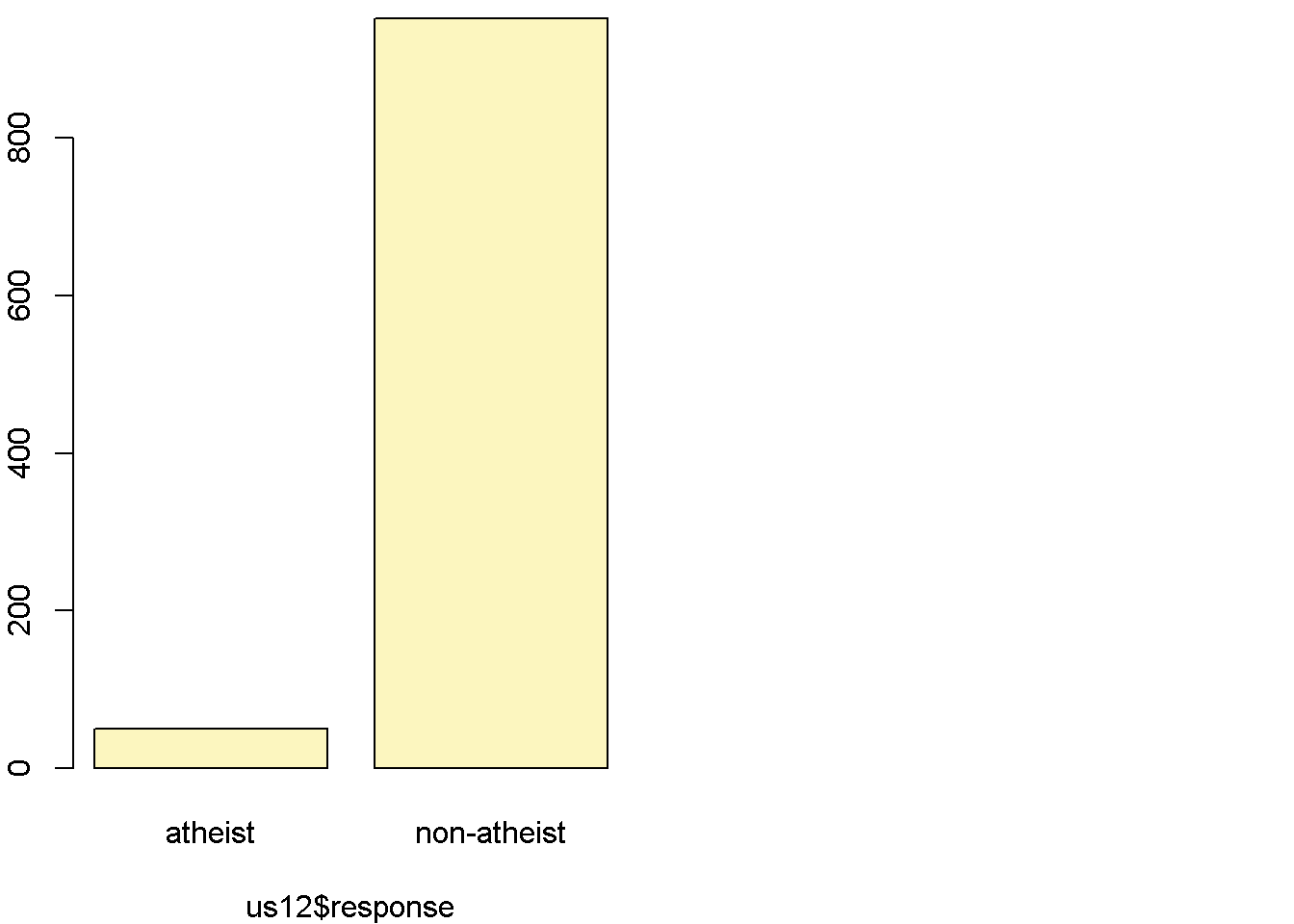## p_hat = 0.0499 ;  n = 1002
## Check conditions: number of successes = 50 ; number of failures = 952
## Standard error = 0.0069
## 95 % Confidence interval = ( 0.0364 , 0.0634 )

### Exercise 6. Based on the R output, what is the margin of error for the estimate of the proportion of the proportion of atheists in US in 2012?

margin of error <- 1.96*0.0069
margin of error
##  0.013524

### Exercise 7. Using the inference function, calculate confidence intervals for the proportion of atheists in 2012 in two other countries of your choice, and report the associated margins of error. Be sure to note whether the conditions for inference are met. It may be helpful to create new data sets for each of the two countries first, and then use these data sets in the inference function to construct the confidence intervals.

##### Belgium and Hong Kong
Bg12 <- subset(atheism, nationality == "Belgium" & year == "2012")
summary(Bg12$response) ## atheist non-atheist ## 42 485 inference(Bg12$response, est = "proportion", type = "ci", method = "theoretical", success = "atheist")
## Single proportion -- success: atheist
## Summary statistics: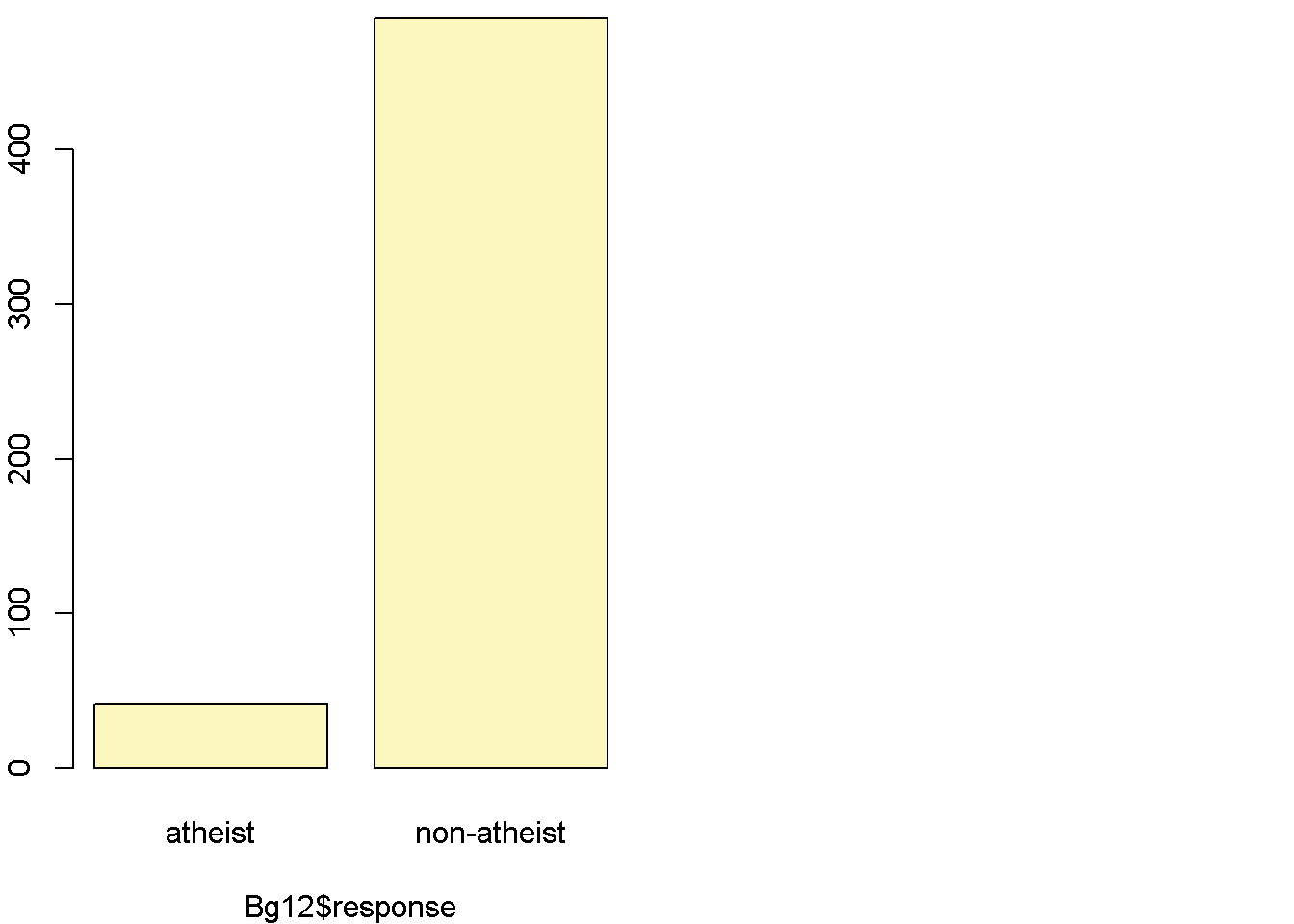## p_hat = 0.0797 ;  n = 527
## Check conditions: number of successes = 42 ; number of failures = 485
## Standard error = 0.0118
## 95 % Confidence interval = ( 0.0566 , 0.1028 )
margin of error<- 1.96*0.0118
margin of error
##  0.023128
HK12 <- subset(atheism, nationality == "Hong Kong" & year == "2012")
summary(HK12$response) ## atheist non-atheist ## 45 455 inference(HK12$response, est = "proportion", type = "ci", method = "theoretical", success = "atheist")
## Single proportion -- success: atheist
## Summary statistics: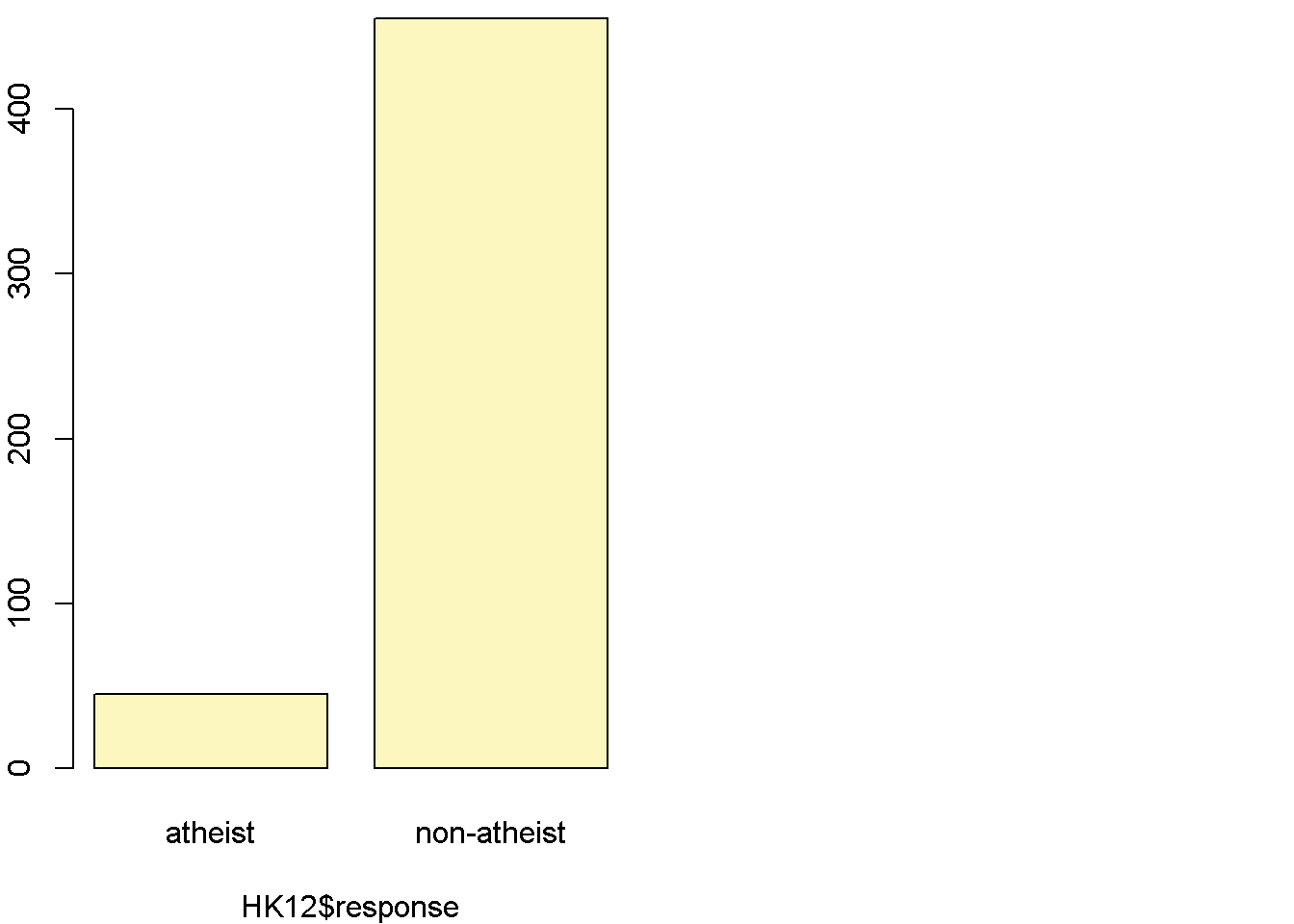## p_hat = 0.09 ;  n = 500
## Check conditions: number of successes = 45 ; number of failures = 455
## Standard error = 0.0128
## 95 % Confidence interval = ( 0.0649 , 0.1151 )
margin of error<- 1.96*0.0128
margin of error
##  0.025088

#### Both countries have met the conditions for constructing confidence interval Belgium’s 95 % Confidence interval = (0.0566, 0.1028) with margin of error of 0.0231. Hong Kong’s 95 % Confidence interval = (0.0649, 0.1151) with margin of error of 0.0251.

##How does the proportion affect the margin of error?

n <- 1000
p <- seq(0, 1, 0.01)
me <- 2 * sqrt(p * (1 - p)/n)
plot(me ~ p, ylab = "Margin of Error", xlab = "Population Proportion")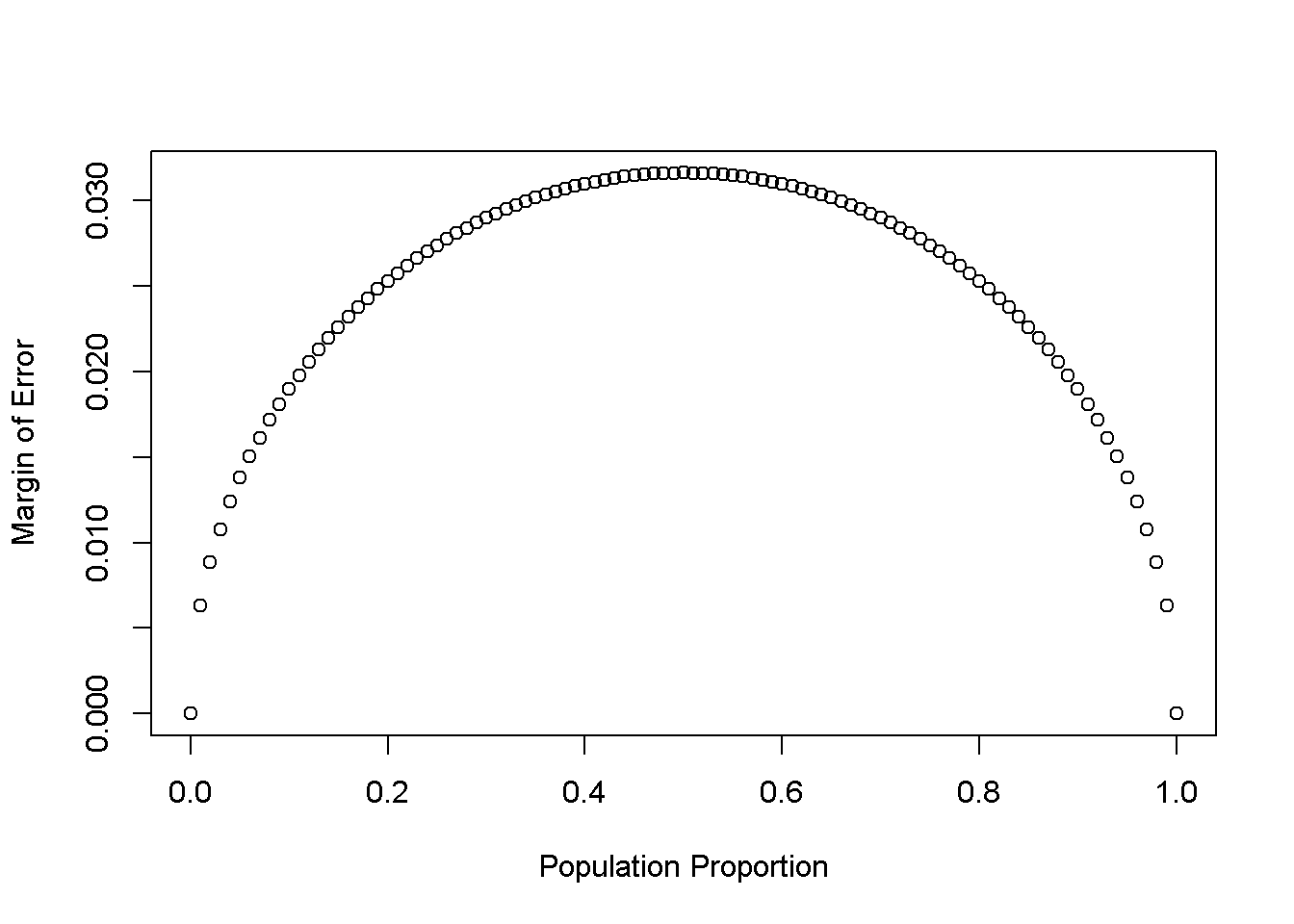### Exercise 8.Describe the relationship between p and me.

#### The plot follows an upside down parabola shape. The margin of error is at highest when the population proportion is at 50%. As the population proportion moves to either extreme ends of 0 or 1, margin of error decreases.

##Success-failure condition

p <- 0.1
n <- 1040
p_hats <- rep(0, 5000)

for(i in 1:5000){
samp <- sample(c("atheist", "non_atheist"), n, replace = TRUE, prob = c(p, 1-p))
p_hats[i] <- sum(samp == "atheist")/n
}

hist(p_hats, main = "p = 0.1, n = 1040", xlim = c(0, 0.18))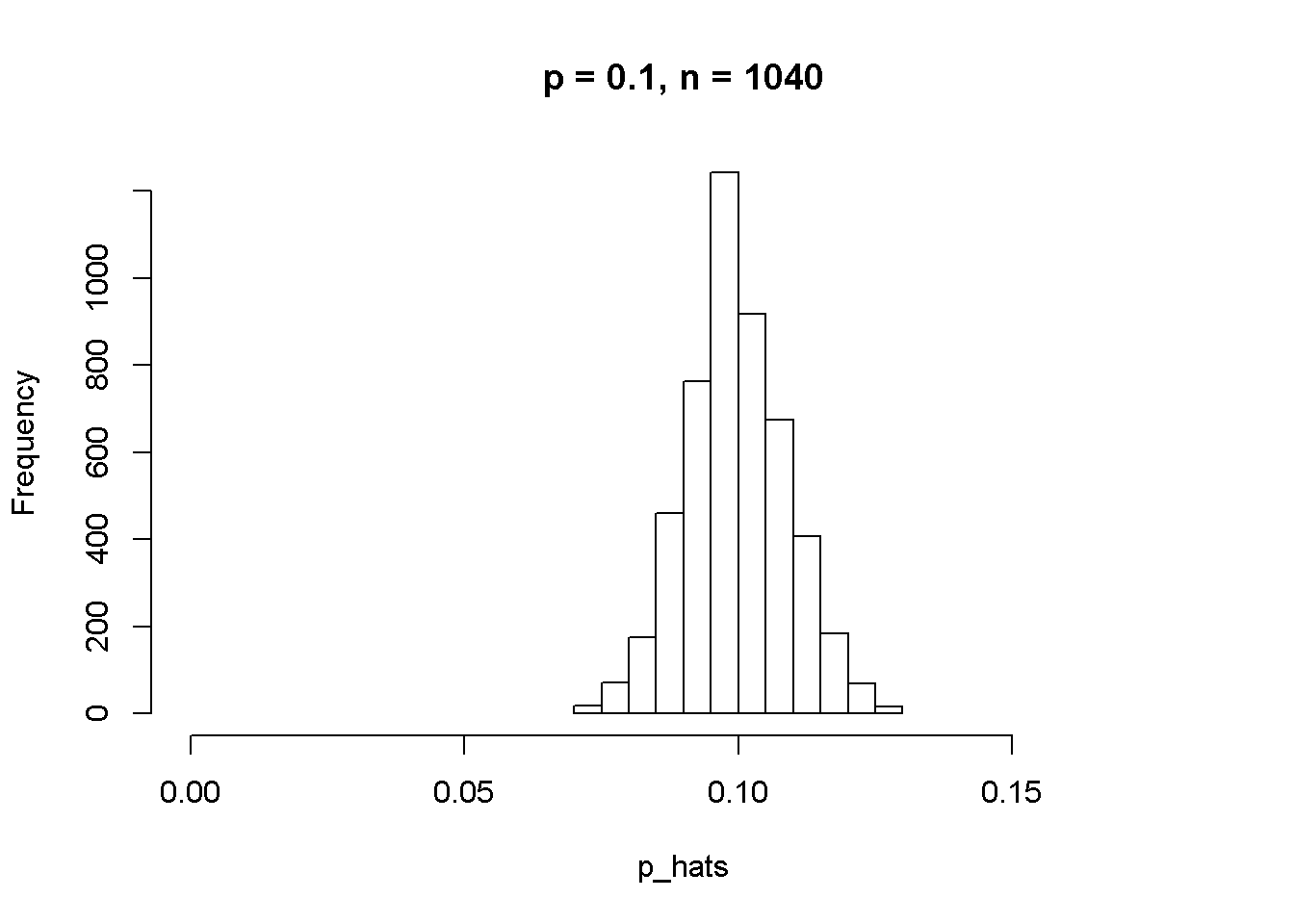### Exercise 9. Describe the sampling distribution of sample proportions at n=1040 and p=0.1. Be sure to note the center, spread, and shape.

summary(p_hats)
##    Min. 1st Qu.  Median    Mean 3rd Qu.    Max.
## 0.07019 0.09327 0.09904 0.09969 0.10577 0.12981

### Exercise 10. Repeat the above simulation three more times but with modified sample sizes and proportions: for n=400 and p=0.1, n=1040 and p=0.02, and n=400 and p=0.02. Plot all four histograms together by running the par(mfrow = c(2, 2)) command before creating the histograms. You may need to expand the plot window to accommodate the larger two-by-two plot. Describe the three new sampling distributions. Based on these limited plots, how does n appear to affect the distribution of p^? How does p affect the sampling distribution?

#### for n=400 and p=0.1

p <- 0.1
n <- 400
p_400.1 <- rep(0, 5000)

for(i in 1:5000){
samp400.1 <- sample(c("atheist", "non_atheist"), n, replace = TRUE, prob = c(p, 1-p))
p_400.1[i] <- sum(samp400.1 == "atheist")/n
}

#### for n=1040 and p=0.2

p <- 0.2
n <- 1040
p_1040.2 <- rep(0, 5000)

for(i in 1:5000){
samp1040.2 <- sample(c("atheist", "non_atheist"), n, replace = TRUE, prob = c(p, 1-p))
p_1040.2[i] <- sum(samp1040.2 == "atheist")/n
}  

#### for n=400 and p=0.2

p <- 0.2
n <- 400
p_400.2 <- rep(0, 5000)

for(i in 1:5000){
samp400.2 <- sample(c("atheist", "non_atheist"), n, replace = TRUE, prob = c(p, 1-p))
p_400.2[i] <- sum(samp400.2 == "atheist")/n
}  
par(mfrow = c(2, 2))
hist(p_hats, main = "p = 0.1, n = 1040", xlim = c(0, 0.18))
hist(p_400.1, main = "p = 0.1, n = 400", xlim = c(0.05, 0.2))
hist(p_1040.2, main = "p = 0.2, n = 1040", xlim = c(0.15, 0.3))
hist(p_400.2, main = "p = 0.2, n = 400", xlim = c(0.1, 0.3))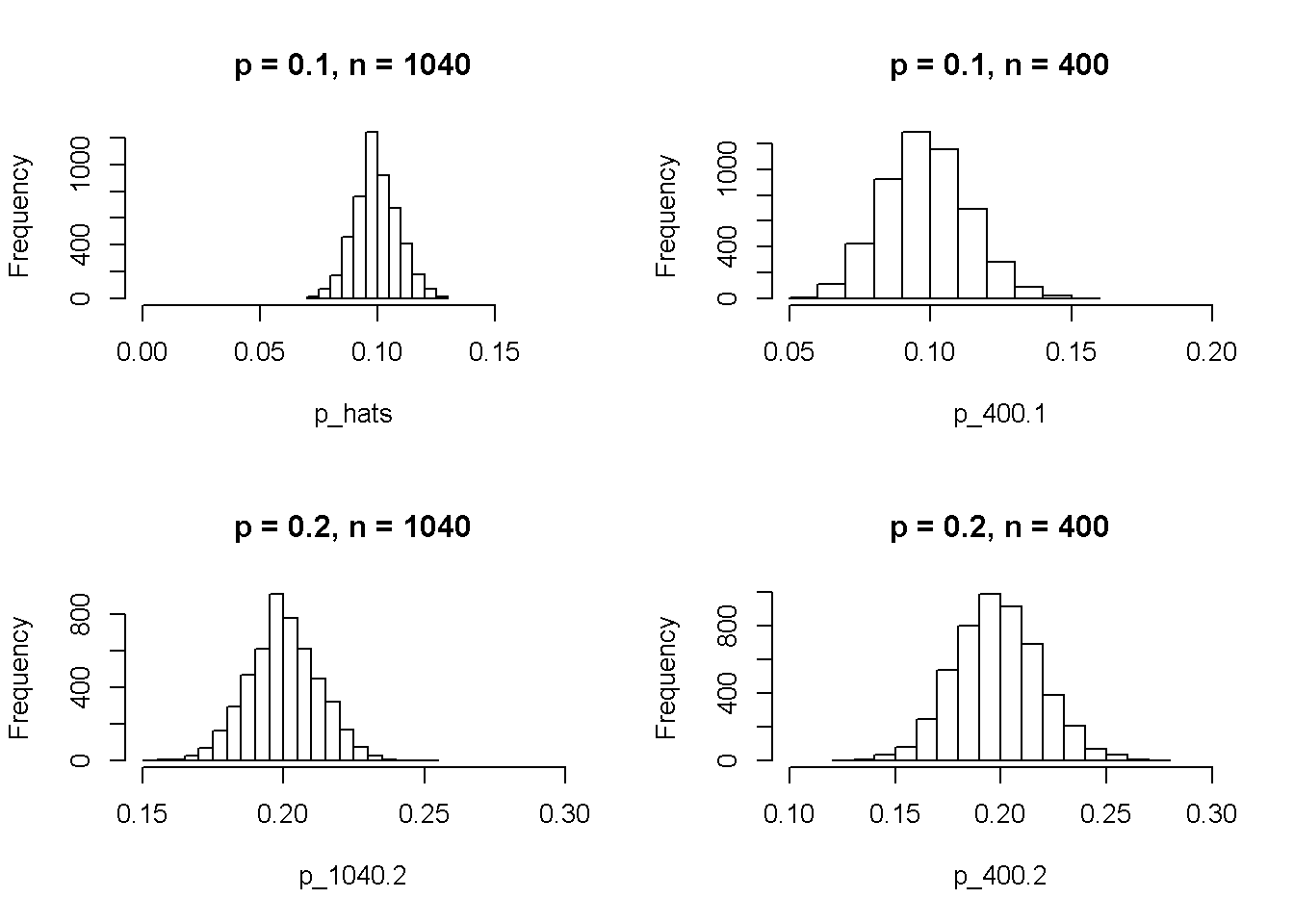### Exercise 11.If you refer to Table 6, you’ll find that Australia has a sample proportion of 0.1 on a sample size of 1040, and that Ecuador has a sample proportion of 0.02 on 400 subjects. Let’s suppose for this exercise that these point estimates are actually the truth. Then given the shape of their respective sampling distributions, do you think it is sensible to proceed with inference and report margin of errors, as the reports does?

#### Australia’s success and failure condition has been met because they are greater than 10 because np=10.4, So it is sensible to proceed with inference and report margin of errors. However, in the case for Ecuador, it did not meet the requirement for success-failure condition because np = 8, which is less than 10. So Ecuador dataset should not proceed to inference and margin of errors as the reports did.

0.01*1040
##  10.4
(1-0.01)*1040
##  1029.6
0.02*400
##  8
(1-0.02)*400
##  392